# Methods and formulas for 1-Sample Wilcoxon

Select the method or formula of your choice.

## Pairwise averages

Pairwise averages (also called Walsh averages) are the means of each possible pair of values in your data set, including the pair of each value with itself.

### Formula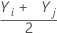= all pairwise averages for i ≤ j.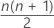= the total number of pairwise averages

### Notation

TermDescription
Yiith value in the data set
Yjjth value in the data set
nsample size

## Estimated median

Let W( 1 )< W( 2 )< ... < W( M ) denote the ordered values of pairwise averages (also called Walsh averages), where M = n(n+1)/2. If M is odd, the estimated median is the middle value. If M is even, the estimated median is the average of the two middle values. Minitab obtains the point estimate of the population median using an algorithm based on Johnson and Mizoguchi (1978)1.
1. D.B. Johnson and T. Mizoguchi (1978). "Selecting the Kth Element in X + Y and X1 + X2 + ... + Xm," SIAM Journal of Computing 7, pp.147-153.

## Wilcoxon statistic

The Wilcoxon statistic is the number of pairwise averages (also called Walsh averages) that are larger than the hypothesized median, plus one half the number of pairwise averages that are equal to the hypothesized median. The Wilcoxon statistic is denoted as W. Minitab obtains the test statistic using an algorithm based on Johnson and Miizoguchi (1978)1.

1. D.B. Johnson and T. Mizoguchi (1978). "Selecting the Kth Element in X + Y and X1 + X2 + ... + Xm," SIAM Journal of Computing 7, 147-153.

## P-value

The Wilcoxon test statistic, W, is the sum of the ranks associated with the observations that exceed the hypothesized median. Minitab calculates the test statistic by using pairwise (Walsh) averages as described in Johnson and Mizoguchi1:

1. The number of observations, N, is reduced by one for each observation that is equal to the hypothesized median. The sample size that results is n.
2. Exclude observations that are equal to the hypothesized median. Calculate n(n + 1) / 2 pairwise Walsh averages (Yi + Yj) / 2 for i ≤ j of the observations.

For large sample sizes, the distribution of W is approximately normal. Specifically: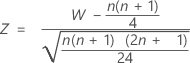is approximately distributed as a normal distribution with a mean of 0 and a standard deviation of 1, N(0,1).

The normal approximation p-value for the three alternative hypotheses uses a continuity correction of 0.5.
Alternative hypothesis P-value
H1: Median > Hypothesized median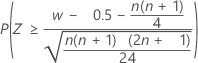H1: Median < Hypothesized median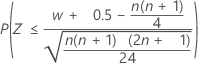H1: Median ≠ Hypothesized median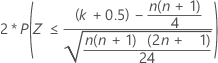### Notation

TermDescription
nthe observed number of data points after the observations that are equal to the hypothesized median value are omitted
Wthe Wilcoxon test statistic
wthe number of Walsh averages that exceed the hypothesized median, plus half of the number of Walsh averages that equal the hypothesized median.
k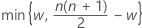1. D.B. Johnson and T. Mizoguchi (1978). "Selecting the Kth Element in X + Y and X1 + X2 + ... + Xm," SIAM Journal of Computing 7, pp.147-153.

## Confidence interval

The confidence interval is the set of values (d) for which the test of H0: median = d is not rejected in favor of H1: median ≠   d, using the confidence level (α = 1 - (percent confidence) / 100). The 1-sample Wilcoxon test does not always achieve the confidence level that you specify because the Wilcoxon statistic is discrete. Because of this, Minitab uses a normal approximation with a continuity correction to calculate the closest achievable confidence level.

By using this site you agree to the use of cookies for analytics and personalized content.  Read our policy